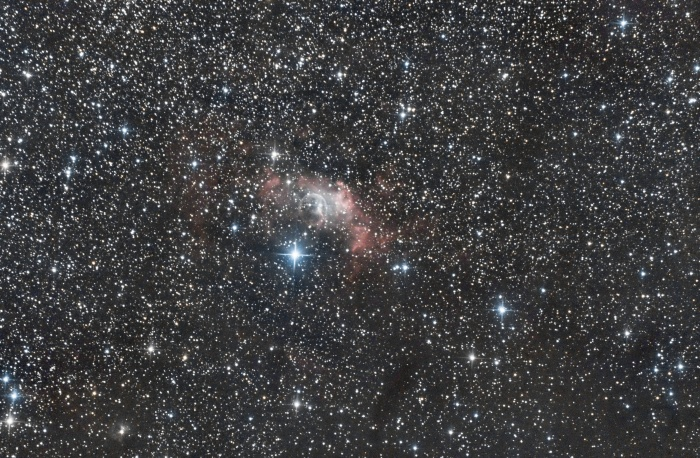Other languages:
English • ‎español • ‎français

# Siril processing tutorial

## Stacking

The final thing to do with Siril is to stack the images. Go to the "stacking" tab, indicate if you want to stack all images, only selected images or the best images regarding the value of FWHM previously computed. Siril proposes several algorithms for stacking computation.

• Sum Stacking

This is the simplest algorithm: each pixel in the stack is summed using 32-bit precision, and the result is normalized to 16-bit. The increase in signal-to-noise ratio (SNR) is proportional to $\sqrt{N}$, where N is the number of images.

• Average Stacking With Rejection
• Sigma Clipping: this is an iterative algorithm which will reject pixels whose distance from median will be farthest than two given values in sigma units ($\sigma_{low}$, $\sigma_{high}$).
• Median Sigma Clipping: this is the same algorithm except than the rejected pixels are replaced by the median value of the stack.
• Winsorized Sigma Clipping: this is very similar to Sigma Clipping method but it uses an algorithm based on Huber's work  .
• Linear Fit Clipping: this is an algorithm developed by Juan Conejero, main developer of PixInsight . It fits the best straight line ($y=ax+b$) of the pixel stack and rejects outliers. This algorithm performs very well with large stacks and images containing sky gradients with differing spatial distributions and orientations.

These algorithms are very efficient to remove satellite/plane tracks.

• Median Stacking

This method is mostly used for dark/flat/offset stacking. The median value of the pixels in the stack is computed for each pixel. As this method should only be used for dark/flat/offset stacking, it does not take into account shifts computed during registration. The increase in SNR is proportional to $0.8\sqrt{N}$.

• Pixel Maximum Stacking

This algorithm is mainly used to construct long exposure star-trails images. Pixels of the image are replaced by pixels at the same coordinates if intensity is greater.

In the case of M8-M20 sequence, we have used on "Winsorized Sigma Clipping Stacking" in "Average stacking with rejection" to remove satellite tracks ($\sigma_{low}=4$ and $\sigma_{high}=2$).

After that, the result is saved in the file named below the buttons, and is displayed in the grey and colour windows. You can adjust levels if you want to see it better, or use the different display mode. In our example the file is the stack result of all files, i.e., 119 files.

The images above picture the result in Siril using the Histogram Equalization rendering mode. Note the improvement of the signal-to-noise ratio regarding the result given for one frame in the previous step (take a look to the sigma value). The increase in SNR is of $38/3.9 = 9.7 \approx \sqrt{119} = 10.9$

Here, comparison between the same crop of calibrated single frame and stacked result.

Now should start the process of the image with crop, background extraction (to remove gradient), and some other processes to enhance your image. To see processes available in Siril please visit this page. Here an example of what you can get with Siril:1. Peter J. Huber and E. Ronchetti (2009), Robust Statistics, 2nd Ed., Wiley
2. Juan Conejero, ImageIntegration, Pixinsight Tutorial

End of the processing tutorial. Return to the main documentation page for more illustrated tutorials.